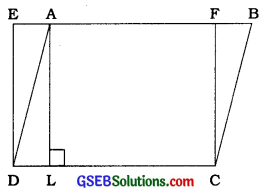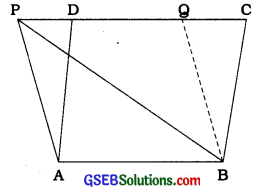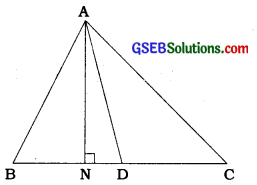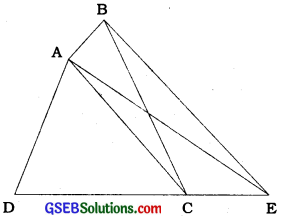# GSEB Class 9 Maths Notes Chapter 9 Quadrilaterals

This GSEB Class 9 Maths Notes Chapter 9 Quadrilaterals covers all the important topics and concepts as mentioned in the chapter.

## Quadrilaterals Class 9 GSEB Notes

Planar region:
The part of the plane enclosed by a simple closed figure is called a planar region corresponding to that figure.

Area of a closed figure:
The magnitude or measure of the planar region cqrresponding to a closed figure is called its area. The magnitude or measure is always expressed with the help of a positive real number (in some units).

Areas of congruent figures:

• If two figures A and B are congruent, they must have equal areas. However, the -converse of this statement is not true. In other words, two figure having equal areas need not be congruent.
• Symbol for the area of a figure: Area of figure A is denoted as ar(A) symbolically.

Properties of area of figures:

• If A and B are two congruent figures, then ar (A) = ar (B).
• If a planar region formed by a figure T is made up of two non-overlapping planar regions formed by figures P and Q, then ar (T) = ar (P) + ar (Q).

Figures on the same base and between , the same parallels:
Two figures are said to be on the same base and between the same parallels, if they have a common base (side) and the vertices (or the vertex) opposite to the common base of each figure lie on a line parallel to the base.
Note: Out of two parallel lines, one line must contain the common base.

Parallelograms on the same base and between the same parallels:

• Theorem 9.1: Parallelograms on the same base (or equal bases) and between the same parallels are equal In area.
• The area of a parallelogram Is the product of Its base and the altitude corresponding to that base.
• Parallelograms on the same base (or equal bases) and having equal areas lie between the same parallels, one of which contains the same (or equal) base / s.
• If a parallelogram and a triangle are on the same base and between the same parallels, then the area of the triangle Is half the area of the parallelogram.Example 1:
In the given figure, ABCD is a parallelogram and EFCD is a rectangle. Also, AL ⊥ DC.
Prove that:
(i) ar (ABCD) = ar (EFCD)
(ii) ar(ABCD) = DC × AL(i) As a rectangle Is also a parallelogram, ar (ABCD) = ar(EFCD) (Theorem 9.1)
(II) From above result, ar (ABCD) = ar (EFCD) = DC × FC
(Area of the rectangle = length × breadth) …… (1)
Since, AL ⊥ DC. AFCL is also a rectangle.
∴ AL = FC … …(2)
Hence, ar(ABCD) = DC × AL [From (1) and (2)]

Example 2:
If a triangle and a parallelogram are on the same base and between the same parallels, then prove that the area of the triangle is equal to half the area of the parallelogram.
Let, ABP and parallelogram ABCD be on the same base AB and between the same parallels AB and PC (See the given figure).Draw BQ ∥ AP to obtain another parallelogram ABQR Now, parallelograms ABQP and ABCD are on the same base AB and between the same parallels AB and PC.
∴ ar(ABQP) = ar(ABCD) (Theorem 9.1) ………… (1)
But, ∆PAB ≅ ∆BQP
(Diagonal PB divides parallelogram ABQP into two congruent triangles.)

∴ ar(PAB)=ar(BQP) …………(2)
∴ ar(PAB)= $$\frac{1}{2}$$ar(ABQP) [From (2)] ………….. (3)
Hence, ar (PAB) = $$\frac{1}{2}$$ar (ABCD) [From (1) and (3))

Triangles on the same base and between the same parallels :
Theorem 9.2:
Two triangles on the same base (or equal bases) and between the same parallels are equal in area.

Area of a triangle:
The area of a triangle is half the product of its base (or any side) and the corresponding altitude (or height).

Theorem 9.3:
Two triangles having the same base (or equal bases) and equal areas lie between the same parallels, one of which contains the base (or bases).

Property of a median of a triangle:
Any median of a triangle divides the triangle into two triangles of equal area.

Example 1:
Show that a median of a triangle divides it into two triangles of equal areas.
Let, ABC be a triangle and let AD be one of its medians (see the given figure).Since the formula for area involves altitude, let us draw AN ⊥ BC.
Now, ar (ABD) = $$\frac{1}{2}$$ × base × altitude (of ∆ ABD)
= $$\frac{1}{2}$$ × BD × AN
= $$\frac{1}{2}$$ × CD × AN (∵ BD = CD)
= $$\frac{1}{2}$$ × base × altitude (of ∆ACD) = ar (ACD)Example 2:
In the given figure, ABCD is a quadrilateral and BE ∥ AC and also BE meets DC produced at E. Show that area of ∆ADE is equal to the area of the quadrilateral ABCD.